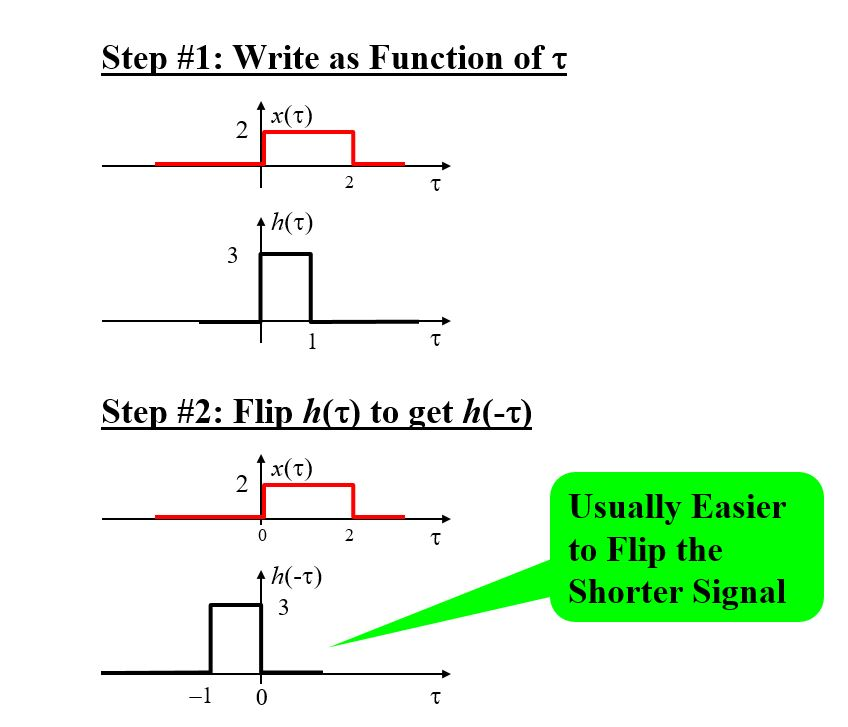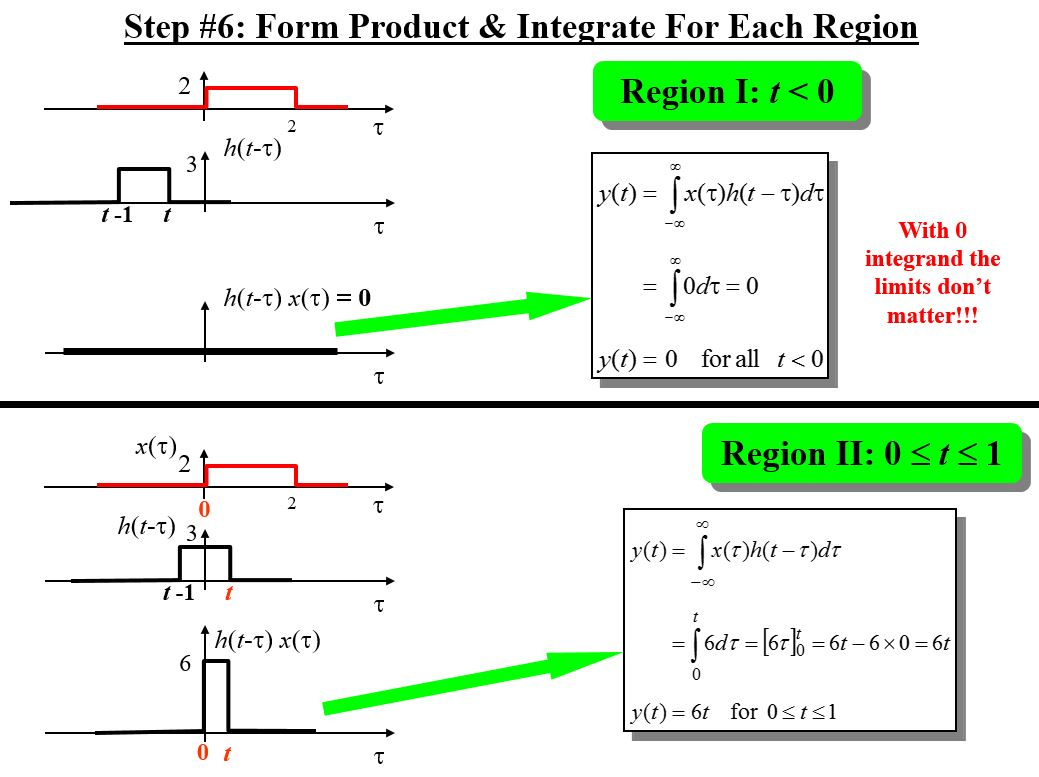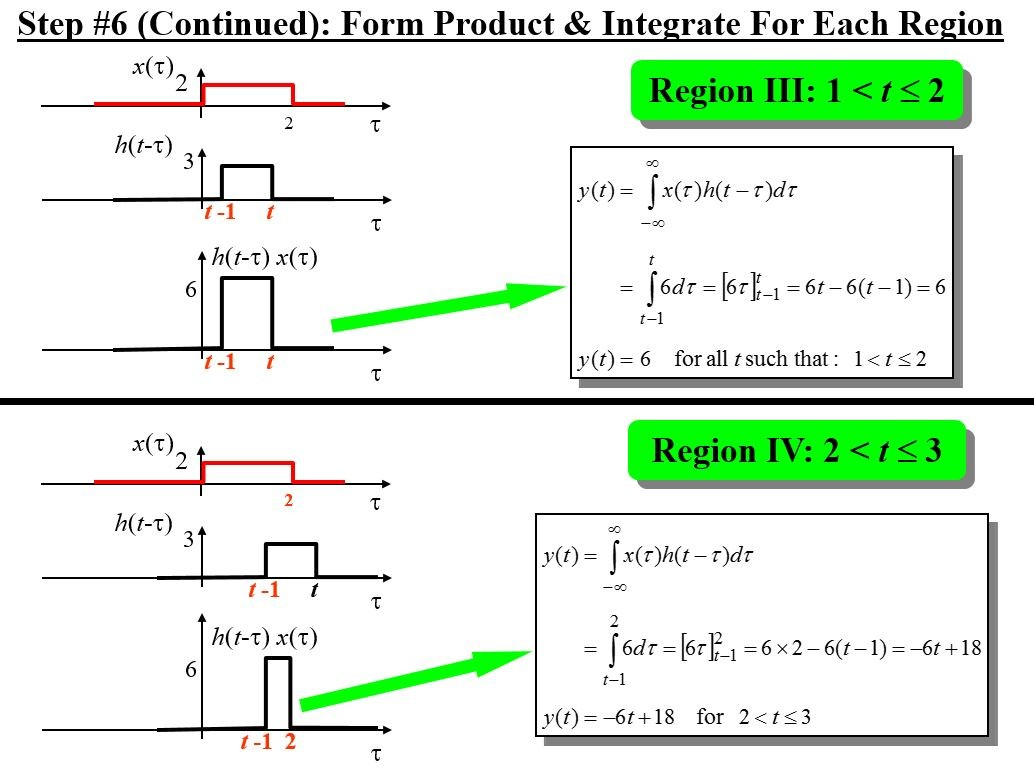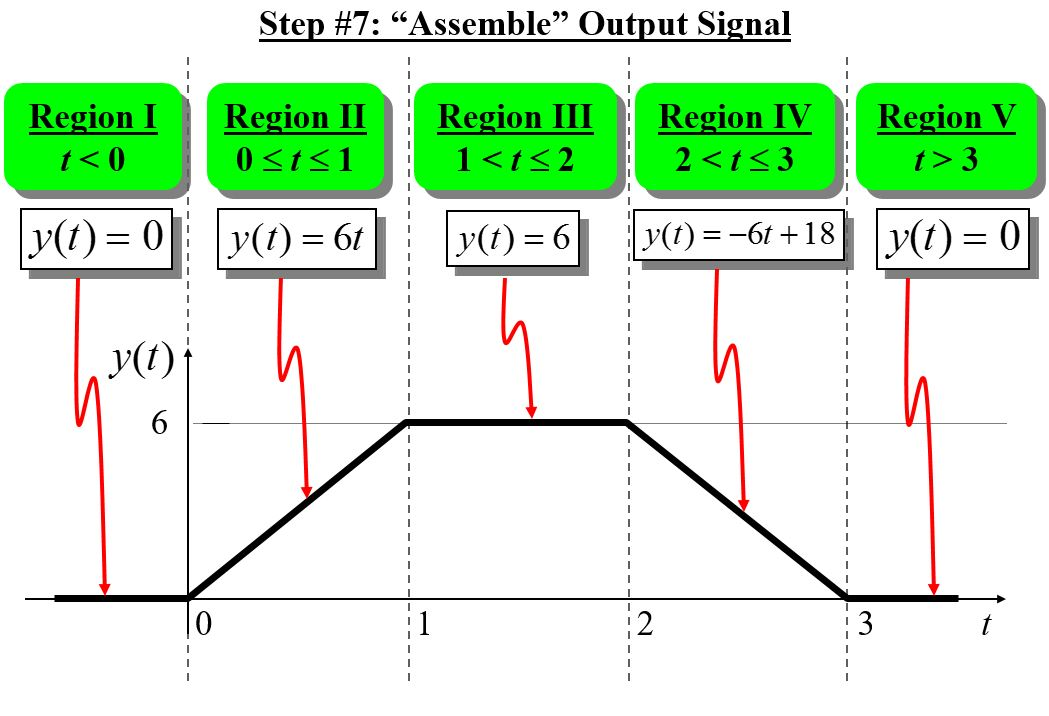Home / Signals and Systems / Continuous Time Graphical Convolution Example

Continuous Time Graphical Convolution Example

Want create site? Find Free WordPress Themes and plugins.

This is the continuation of the PREVIOUS TUTORIAL.

Steps for Graphical Convolution

1. First of all re-write the signals as functions of τ: x(τ) and h(τ)
1. Flip one of the signals around t = 0 to get either x(-τ) or h(-τ)
• Best practice is to flip the signal with shorter interval
• We will flip h(τ) to get h(-τ) throughout the steps
1. Determine Edges of the flipped signal
• Determine the left-hand edge τ-value of h(-τ): say τL,0
• Determine the right-hand edge τ-value of h(-τ): say τR,0
1. Shifting h(-τ) by a random value of t to obtain h(t-τ) and get its edges
• Determine the left-hand edge τ-value of h(t-τ) as a function of t: say τL,t

Noteworthy: It will forever be…τL,t = t + τL,0

• Determine the right-hand edge τ-value of h(t-τ) as a function of t: say τR,t

Noteworthy: It will forever be…τR,t = t + τR,0

1. Find out Regions of τ-Overlap
•  Determine intervals of t over which the product x(τ) h(t-τ) possesses a single unique mathematical form in terms of τ
1. For Each Particular Region: develop the Product x(τ) h(t-τ) and Integrate
• Develop the product x(τ) h(t-τ)
• Determine the boundaries of Integration by determining the interval of τ over which the mathematical product is nonzero
•  Determine by discovering where the bounds of x(τ) and h(t-τ) lie down
•  Call back that the bounds of h(t-τ) are τL,t and τR,t , which frequently depend upon the value of t
• Integrate the product x(τ) h(t-τ) over the boundaries determined in 6b
1. “Put Together” the output from the output time-sections for each of the region important:
• DO NOT add together all the sections
• Specify the output in “piecewise” mannerThis example is provided in collaboration with Prof. Mark L. Fowler, Binghamton University.

Did you find apk for android? You can find new Free Android Games and apps.